Assertion & Reason Test: Triangles - 1

# Assertion & Reason Test: Triangles - 1 - Class 10

Test Description

## 10 Questions MCQ Test Mathematics (Maths) Class 10 - Assertion & Reason Test: Triangles - 1

Assertion & Reason Test: Triangles - 1 for Class 10 2023 is part of Mathematics (Maths) Class 10 preparation. The Assertion & Reason Test: Triangles - 1 questions and answers have been prepared according to the Class 10 exam syllabus.The Assertion & Reason Test: Triangles - 1 MCQs are made for Class 10 2023 Exam. Find important definitions, questions, notes, meanings, examples, exercises, MCQs and online tests for Assertion & Reason Test: Triangles - 1 below.
Solutions of Assertion & Reason Test: Triangles - 1 questions in English are available as part of our Mathematics (Maths) Class 10 for Class 10 & Assertion & Reason Test: Triangles - 1 solutions in Hindi for Mathematics (Maths) Class 10 course. Download more important topics, notes, lectures and mock test series for Class 10 Exam by signing up for free. Attempt Assertion & Reason Test: Triangles - 1 | 10 questions in 20 minutes | Mock test for Class 10 preparation | Free important questions MCQ to study Mathematics (Maths) Class 10 for Class 10 Exam | Download free PDF with solutions
 1 Crore+ students have signed up on EduRev. Have you?
Assertion & Reason Test: Triangles - 1 - Question 1

### Direction: In the following questions, A statement of Assertion (A) is followed by a statement of Reason (R). Mark the correct choice as. Assertion (A): In an equilateral triangle of side 3√3 cm, then the length of the altitude is 4.5 cm. Reason (R): If a ladder 10 cm long reaches a window 8 m above the ground, then the distance of the foot of the ladder from the base of the wall is 6 m.

Detailed Solution for Assertion & Reason Test: Triangles - 1 - Question 1

In case of assertion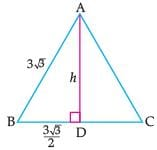ΔABD, ∠D = 90°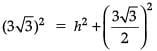or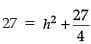or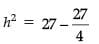or, h2 = 81/4

∴ h = 9/2 = 4.5 m

∴ Assertion is correct.

In case of reason: Let BC be the height of the window above the ground and AC be a ladder.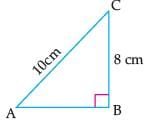Here, BC = 8 cm and AC = 10 cm

∴ In right angled triangle ABC, AC2 = AB2 + BC2

(By using Pythagoras Theorem)

⇒ (10)2 = AB2 + (8)2

⇒ AB2 = 100 – 64

= 36

⇒ AB = 6 m.

∴ Reason is correct.

Hence, both assertion and reason are correct but reason is not the correct explanation for assertion.

Assertion & Reason Test: Triangles - 1 - Question 2

### Direction: In the following questions, A statement of Assertion (A) is followed by a statement of Reason (R). Mark the correct choice as.Assertion : In the ΔABC , AB = 24 cm , BC = 10 cm and AC = 26 cm , then ΔABC is a right angle triangle.Reason : If in two triangles, their corresponding angles are equal, then the triangles are similar.

Detailed Solution for Assertion & Reason Test: Triangles - 1 - Question 2
We have, AB2 + BC2 = (24)2 + (10)2

= 576 + 100 = 676 = 2

AB2 + BC2 = AC2

ABC is a right angled triangle.

Also, two triangle are similar if their corresponding angles are equal.

So, both A and R are correct but R does not explain A.

Assertion & Reason Test: Triangles - 1 - Question 3

### Direction: In the following questions, A statement of Assertion (A) is followed by a statement of Reason (R). Mark the correct choice as.Assertion : The length of the side of a square whose diagonal is 16 cm, is 8√2 cmReason : In a right triangle, the square of the hypotenuse is equal to the sum of the squares of the other two sides.

Detailed Solution for Assertion & Reason Test: Triangles - 1 - Question 3
We know that In a right triangle, the square of the hypotenuse is equal to the sum of the squares of the other two sides. This is Pythagoras theorem. So, Reason is correct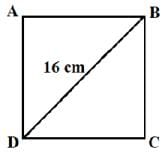Let the side of square be x cm.

In ∆ABD, by Pythagoras theorem, we have BD2 = AB2 + AD2

⇒ 162 = x2 + x2

⇒ 2x2 = 256 ⇒ x2 = 128 ⇒ x = 8√2 cm

So, Assertion is also correct.

Assertion & Reason Test: Triangles - 1 - Question 4

Direction: In the following questions, A statement of Assertion (A) is followed by a statement of Reason (R). Mark the correct choice as.

Assertion (A): Corresponding sides of two similar triangles are in the ratio of 2 : 3. If the area of the smaller triangle is 48 cm2, then the area of the larger triangle is 108 cm2.

Reason (R): If D is a point on the side BC of a triangle ABC such that ∠ADC = ∠BAC, then CA2 = CB × CD.

Detailed Solution for Assertion & Reason Test: Triangles - 1 - Question 4
In case of assertion

Let ΔABC and ΔDEF are two similar triangles.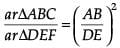Given that ar ΔABC = 48 cm2. Then,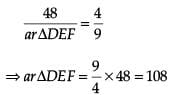Thus, the area of larger triangle is 108 cm2.

∴ Assertion is correct.

In case of reason: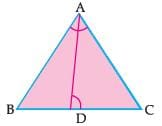∠BCA= ∠ACD [Common angle]

By AA similarity, ΔBAC ~ ΔADC

Thus,

CA/CD = BC/CA

∴ Reason is correct.

Hence, both assertion and reason are correct but reason is not the correct explanation for assertion.

Assertion & Reason Test: Triangles - 1 - Question 5

Direction: In the following questions, A statement of Assertion (A) is followed by a statement of Reason (R). Mark the correct choice as.

Assertion : ABC and DEF are two similar triangles such that BC = 4 cm , EF = 5 cm and area of ΔABC = 64 cm2 , then area of ΔDEF = 100 cm2.

Reason : The areas of two similar triangles are in the ratio of the squares of teh corresponding altitudes.

Detailed Solution for Assertion & Reason Test: Triangles - 1 - Question 5
Reason is true. [standard result]

For Assertion, since ΔABC ∼ ΔDEF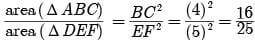(ratio of areas of two similar Δs is equal to the ratio of the squares of corresponding sides)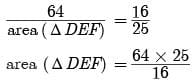= 4 x 25 = 100 cm2

Assertion is true. But Reason is not correct explanation for Assertion.

Assertion & Reason Test: Triangles - 1 - Question 6

Direction: In the following questions, A statement of Assertion (A) is followed by a statement of Reason (R). Mark the correct choice as.

Assertion : The areas of two similar triangles ABC and PQR are in the ratio 9 :16. If BC = 4.5 cm, then the length of QR is 6 cm.

Reason : The ratio of the areas of two similar triangles is equal to the ratio of their corresponding sides.

Detailed Solution for Assertion & Reason Test: Triangles - 1 - Question 6
We know that the ratio of the areas of two similar triangles is equal to the square of the ratio of their corresponding sides.

So, Reason is not correct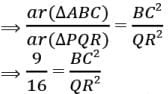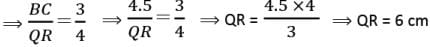So, Assertion is correct

Assertion & Reason Test: Triangles - 1 - Question 7

Direction: In the following questions, A statement of Assertion (A) is followed by a statement of Reason (R). Mark the correct choice as.

Assertion (A): If D is a point on side QR of ΔPQR such that PD ⊥ QR, then ΔPQD ~ ΔRPD.

Reason (R): In the figure given below, if ∠D = ∠C then ΔADE ~ ΔACB.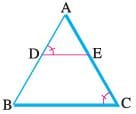Detailed Solution for Assertion & Reason Test: Triangles - 1 - Question 7
In case of assertion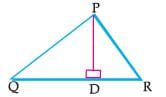In ΔPQD and ΔRPD, ∠PDQ = ∠PDR= 90°.

There is no other information to be similar.

Thus, it is not it will be correct to say that ΔPQD ~ ΔRPD.

∴ Assertion is incorrect.

In case of reason:

In ΔADE and ΔACB, we have

∠DAE= ∠CAB [Common angle]

By AA similarity, we get ΔADE ~ ΔACB.

∴ Reason is correct

Hence, assertion is incorrect but reason is correct

Assertion & Reason Test: Triangles - 1 - Question 8

Direction: In the following questions, A statement of Assertion (A) is followed by a statement of Reason (R). Mark the correct choice as.

Assertion : ABC is an isosceles, right triangle, right angled at C . Then AB2 = 3AC2

Reason : In an isosceles triangle ABC if AC = BC and AB2 = 2AC2 , then ∠C = 90°

Detailed Solution for Assertion & Reason Test: Triangles - 1 - Question 8
If Assertion is incorrect, but Reason is correct.

In right angled ΔABC ,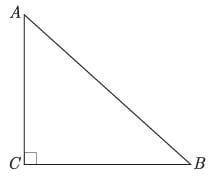AB2 = AC2 + BC2(By Pythagoras Theorem)

= AC2 + AC2 [BC = AC]

= 2AC2AB2 = 2AC2

Assertion is false.

Again since, AB2 = 2AC2

= AC2 + AC2 = AC2 + BC2 (AC = BC given)

∠ = 90°

(By converse of Pythagoras Theorem)Reason is true.

Assertion & Reason Test: Triangles - 1 - Question 9

Direction: In the following questions, A statement of Assertion (A) is followed by a statement of Reason (R). Mark the correct choice as.

Assertion : In ∆ABC , AB = 6√3, AC = 12 cm and BC = 6cm then ∠B = 90°.

Reason : If in a triangle, square of one side is equal to the sum of the squares of the other two sides, then the angle opposite the first side is a right angle.

Detailed Solution for Assertion & Reason Test: Triangles - 1 - Question 9
We know that If in a triangle, square of one side is equal to the sum of the squares of the other two sides, then the angle opposite the first side is a right angle. This is converse of Pythagoras theorem.

So, Reason is correct

Now, AB2 = (6√3)2 = 108

AC2 = 122 = 144

and BC2 = 62 = 36

∴ 2 = 2 + 2

By converse of Pythagoras theorem, ∠B = 90°

So, Assertion is also correct.

Assertion & Reason Test: Triangles - 1 - Question 10

Direction: In the following questions, A statement of Assertion (A) is followed by a statement of Reason (R). Mark the correct choice as.

Assertion (A): If in two right triangles, one of the acute angles of one triangle is equal to an acute angle of the other triangle, then triangles will be similar.

Reason (R): If the ratio of the corresponding altitudes of two similar triangles is 3/5, then the

ratio of their areas is 6/5.

Detailed Solution for Assertion & Reason Test: Triangles - 1 - Question 10
In case of assertion: In the given two right triangles, both have equal right angles and one of the acute angles of one triangle is equal to an acute angle of the other triangle.

Thus, by AA similarity, the given two triangles are similar.

∴ Assertion is correct.

In case of reason:

We know that the ratio of the areas of two similar triangles is the square of the ratio of the corresponding altitudes of two similar triangles.

Thus, the ratio of the areas of two similar triangles is (3/5)2 = 9/25.

## Mathematics (Maths) Class 10

115 videos|479 docs|129 tests
Information about Assertion & Reason Test: Triangles - 1 Page
In this test you can find the Exam questions for Assertion & Reason Test: Triangles - 1 solved & explained in the simplest way possible. Besides giving Questions and answers for Assertion & Reason Test: Triangles - 1, EduRev gives you an ample number of Online tests for practice

## Mathematics (Maths) Class 10

115 videos|479 docs|129 tests